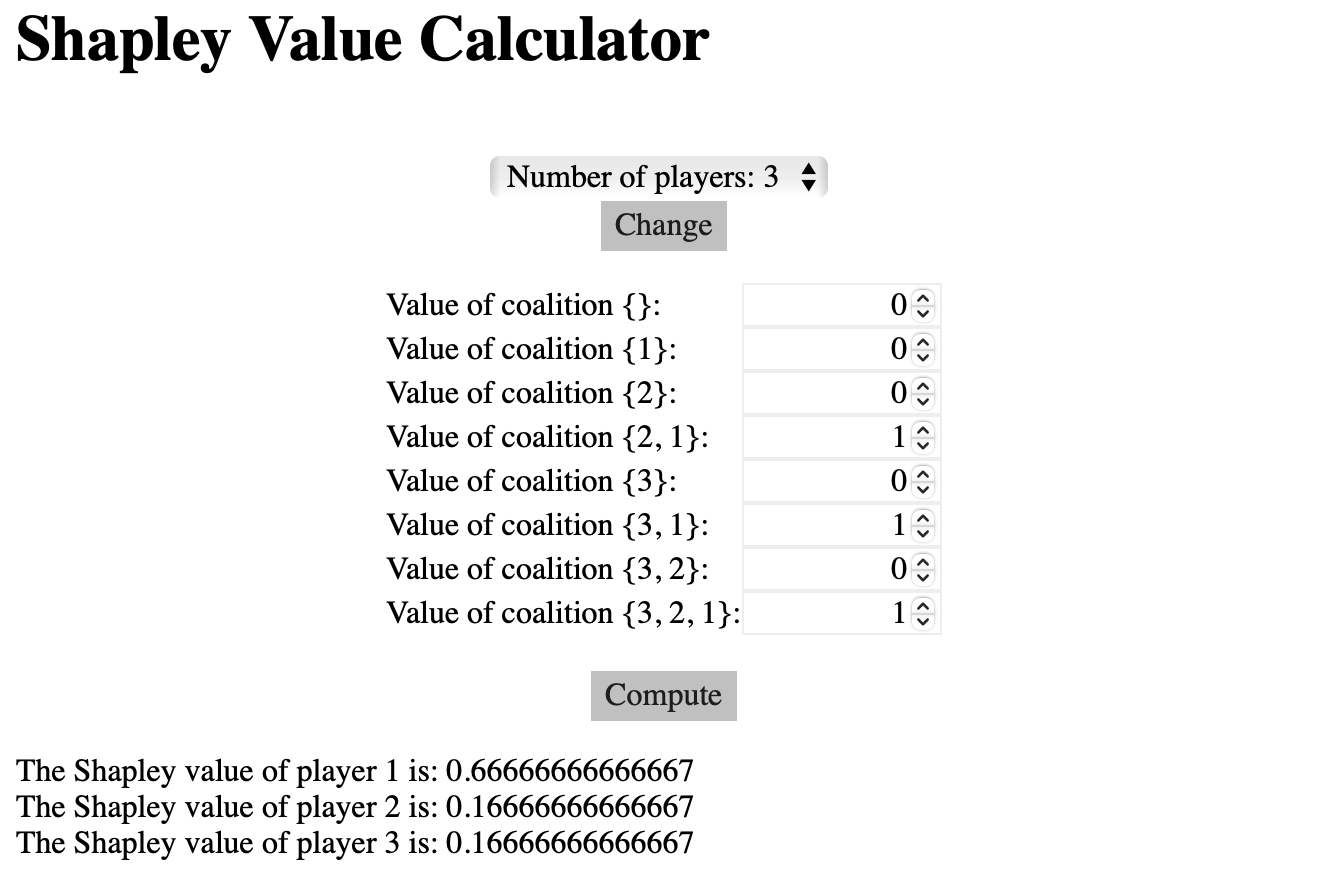# Partnerships and Coalitions

The core game:

Empty core game:
v(AB) = 118
v(BC) = 50
v(AC) = 84
v(ABC) = 121

## Core

Core:
Alternative definition: Set of allocations against which you cannot make an objection

Objection: Let $x$ be a proposed allocation. Let $k \neq l$ be two players in the coalition.

Objection of $k$ against $l$ is a pair $(C,y)$:
• 1. $C \subseteq N$ s.t. $k \in C, l \notin C$ [I am in the subgroup, you are not]
• 2. $\sum_i y_i = v(C) ; y_i > x_i \ \forall i \in C$ [If we kick you out, we are all better off]

Counter objection: $x$ is the originally proposed allocation, $k$ objects to $l$ -- $(C,y)$'s counter objection $l$ to $k$ is a pair $(D,z)$
• 1. $D \subseteq N$ s.t. $l \in D, k \notin D$
• 2. $\sum_{i \in D} z_i = v(D)$ s.t. (a) $z_i > x_i \ \forall i \notin C$ and (b) $z_i > y_i \ \forall i \in C$

Justified objection: An objection that has no counter objection
Bargaining set: That allocation that has no justified objection.

Nucleolus: Most fair allocation among the stable allocations. Nucleolus exists in the bargaining set. If core is not empty, nucleolus will be at the center of the core.

## Exercise 1

Find an unjustified objection to the allocation with counter objection: (79,39,3). Also,find a justified objection.

v(AB) = 118
v(BC) = 50
v(AC) = 84
v(ABC) = 121

Nucleolus solution: $(74\frac{1}{3},40\frac{1}{3},6\frac{1}{3})$ there will always be a counter objection.

Core is allocations with no objection. Core can be unfair

## Exercise 2: Glove game

A Left
B Right
C Right

v(A) = 0
v(B) = 0
v(C) = 0
v(AB) = 1
V(BC) = 0
V(AC) = 1
v(ABC) = 1

Q. Is the allocation in core: (1,0,0) : Yes
Q. Is this a core allocation: (0,1,0) : No

(1,0,0) is the only stable solution -- core is non empty

Q. Where is nucleolus? Nucleolus is inside core. Core has one solution. This allocation (1,0,0) is the nucleolus.

Q. Is (1,0,0) fair? Nope. Competition in B and C is leading to A to squeeze out from them. Prisoners Dilemma/ Gaming nature between B and C is hurting both of them.

## Least Core

Core: $x(s) \leq c(s)$

Least core:
unhappiness = $u(x) = x(s) - c(s)$
$u(s) <= e$ ; minimize $e$

## Calculating Nucleolus

For a general problem, nucleolus is calculated by iteratively decreasing unhappines after finding least core allocation.

## Shapley Value

(1) All value should be allocated $$\sum_i x_i = v(N)$$
(2) Symmetry: For all $s \subseteq N\\{i,j}$, where $$v(s \cup {i})-v(s) = v(s \cup {j})-v(s)$$ Then, $$x_i = x_j$$
(3) Dummy player property Suppose $$v(s \cup {i}) = v(s) + d \ \forall S \subseteq N\\{i}$$ Then $$x_i = d$$
(4) $w(s) = k \times v(s) \forall s \Rightarrow$ the allocation in $x_i^w = kx_i^v$

Calculating shapely value:

Average of the marginal value player $i$ brings in permutations of players that have been chosen before $i$

$$\text{Shapely Value} = \sum_{S \subseteq N\\{i}} \dfrac{|S|!(n-|S|-1)!}{n!}\left(v(S\cup{i})-v(s)\right)$$

## Exercise 3

Calculate shapely value for glove game:
(1) Hard way -- solve it yourself
(2) Easy way -- shapelyvalue.com## Voting Ideas

How to take group decisions?

Top preference: Voting just the "top choice" is not ideal, votes could divert the existing majority votes to newer/other choices.

Assigning value to each rank (Weighted allocation): Susceptible to change to an irrelevant non-winning choice can impact the winning choice.

Multiple two-way elections A>B, B>C, C>A --> ???

Elimination voting: Collecting all preferences for voting, eliminate who score least for 1st rank and so on. Issue: Person with massive victory could lose if a few people change their votes to prefer the winning person.

Kenneth Arrow-- Any voting protocol between 3 or more alternatives will have one of these flaws:
2. Tactical voting
3. Disctatorship (One person's vote decides everything)

(1) Never decide things by voting A>B, B>C, C>A
(2)Try debate and convince (consensus)

## Exercise 4: UN Security Council

Scenario 1 (Older): Permanent Members |P| = 5, Non-permanent |T| = 6
$$v(S) = 1 if P \subseteq S \suchthat |S| \geq 7, 0\ \text{otherwise}$$

Scenario 2 (Newer): Permanent Members |P| = 5, Non-permanent |T| = 10
$$v(S) = 1 if P \subseteq S, |S| \geq 9, 0\ \text{otherwise}$$

Calculating power of countries using shapely value:

[Scenario 1] All 5 Permanent member + 1 Non Permanent member before you. $$\text{Shapley value of single non permanent member} = 1 \times 5 \times \dfrac{6! \times 4!}{11!} = 0.002$$ Now, calculating power of one permanent member: $$6t + 5p = 1 \Rightarrow p = 0.19$$ Hence, permanent members have 90x power than non permanent members.

[Scenario 2] All 5 Permanent member + 3 Non Permanent member before you. $$\text{Shapley value of single non permanent member} = 1 \times ^9C_3 \times \dfrac{8! \times 6!}{15!} = 0.001865$$ Now, calculating power of one permanent member: $$10t + 5p = 1 \Rightarrow p = 0.19$$ Hence, permanent members have 105x power than non permanent members.

## Elevator game

1. Apartment building with 6 floor, 4 houses per floor
2. 72000 Euros for building a lift
3. Heterogenous factors: (1) Lower floor people might not need it (2)

Absense of value creating strategies. People could overstate/ understate the value. Home Owner's Association could enforce rules.

Q. When do we want the lift?

$v_i$ : Private valuation of the home owners

Construct if $\sum v_i \geq V = 72000$

Q. How to not incentivize lying Eliminating conflict of interest. Example: "How much did others work in the group assignment?" This eliminates the consequences of your answer and what is reported for others.

## Pivot mechanism

Pivotal person Single person whose single high/low valuation changes the entire group's. They are to be penalized for the huge gap between the fair value and their bid.